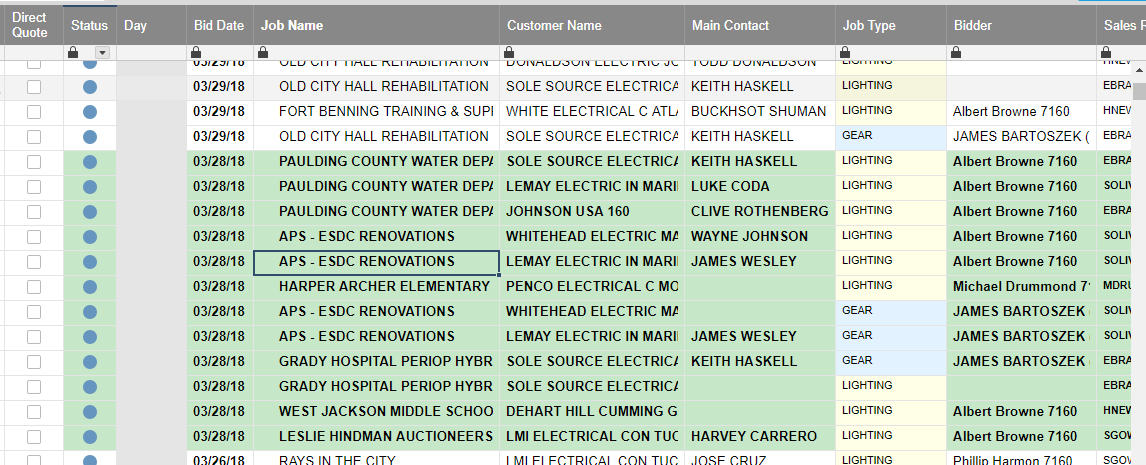# Day of Week instead of short date

Hello,

I would like to have a formula in place that automatically selects a day of week based on the date we paste in the "Bid Date" Column. When 3/26/18 is loaded into [Bid Date] Column it produces "Monday" in the [Day] Column, and so forth... Is this possible?• This is long, but try:

=IF(WEEKDAY(Bid Date1) = 1, "Sunday", IF(WEEKDAY(Bid Date1) = 2, "Monday", IF(WEEKDAY(Bid Date1) = 3, "Tuesday", IF(WEEKDAY(Bid Date1) = 4, "Wednesday", IF(WEEKDAY(Bid Date1) = 5, "Thursday", IF(WEEKDAY(Bid Date1) = 6, "Friday", IF(WEEKDAY(Bid Date1) = 7, "Saturday")))))))

Put that formula in Day1 and then drag it down as needed.

Unfortunately, this is what I got #Unparseable

• Disregard Wade. I realized I needed brackets around [Bid Date]. Works Great!

• I suggest another more clean way:

Create a new sheet let's say called "conversioni" with a conversion table like (sorry italian days...)

then in the data sheet you create a formula

=INDEX({CONVERSIONI: weekday}; MATCH(WEEKDAY([Creato il]@row); {CONVERSIONI: Weeknumber}; 0))

that point at that conversion table and transform your weekday in the word you chose

then you can create a column formula and that's all...

## Help Article Resources

Want to practice working with formulas directly in Smartsheet?

Check out the Formula Handbook template!